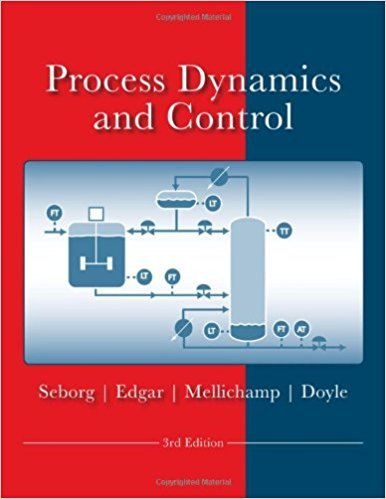×
Get Full Access to Process Dynamics And Control - 3 Edition - Chapter 4 - Problem 4.8
Get Full Access to Process Dynamics And Control - 3 Edition - Chapter 4 - Problem 4.8

×

# A surge tank in Fig. E4.8 is designed with a slotted weirISBN: 9780470128671 148

## Solution for problem 4.8 Chapter 4

Process Dynamics and Control | 3rd Edition

• Textbook Solutions
• 2901 Step-by-step solutions solved by professors and subject experts
• Get 24/7 help from StudySoup virtual teaching assistantsProcess Dynamics and Control | 3rd Edition

4 5 1 270 Reviews
28
5
Problem 4.8

A surge tank in Fig. E4.8 is designed with a slotted weir so that the outflow rate, w, is proportional to the liquid level to the 1.5 power; that is, w = Rh15 where R is a constant. If a single stream enters the tank with flow rate wi, find the transfer function H'(s)!W'(s). Identify the gain and all time constants. Verify units. The cross-sectional area of the tank is A. Density p is constant.

Step-by-Step Solution:

Problem 4.8A surge tank in Fig. E4.8 is designed with a slotted weir so that the outflow rate, w, is 1.5proportional to the liquid level to the 1.5 power; that is, w = Rh where R is a constant. If asingle stream enters the tank with flow rate wi, find the transfer function H'(s)/W'(s). Identifythe gain and all time constants. Verify units. The cross-sectional area of the tank is A.Density p is constant. Step-by-step solution Step 1 of 9 ^Refer to Figure E4.8 from the textbook.Consider the given equation, 1.5 w = Rh ………(1)Where,Outflow rate is represented as w,Constant is represented as R, andHeight of the tank is represented as h.From the figure, the mass balance equation is, d(Ah) = w i w ……….(2) dtWhere,Density is represented as ,Flow rate is represented as w and iCross-sectional area of the tank is represented as A.

Step 2 of 9

Step 3 of 9

##### ISBN: 9780470128671

This textbook survival guide was created for the textbook: Process Dynamics and Control, edition: 3. The full step-by-step solution to problem: 4.8 from chapter: 4 was answered by , our top Engineering and Tech solution expert on 11/15/17, 04:03PM. Since the solution to 4.8 from 4 chapter was answered, more than 895 students have viewed the full step-by-step answer. The answer to “A surge tank in Fig. E4.8 is designed with a slotted weir so that the outflow rate, w, is proportional to the liquid level to the 1.5 power; that is, w = Rh15 where R is a constant. If a single stream enters the tank with flow rate wi, find the transfer function H'(s)!W'(s). Identify the gain and all time constants. Verify units. The cross-sectional area of the tank is A. Density p is constant.” is broken down into a number of easy to follow steps, and 75 words. Process Dynamics and Control was written by and is associated to the ISBN: 9780470128671. This full solution covers the following key subjects: tank, constant, rate, outflow, Density. This expansive textbook survival guide covers 24 chapters, and 420 solutions.

Unlock Textbook Solution